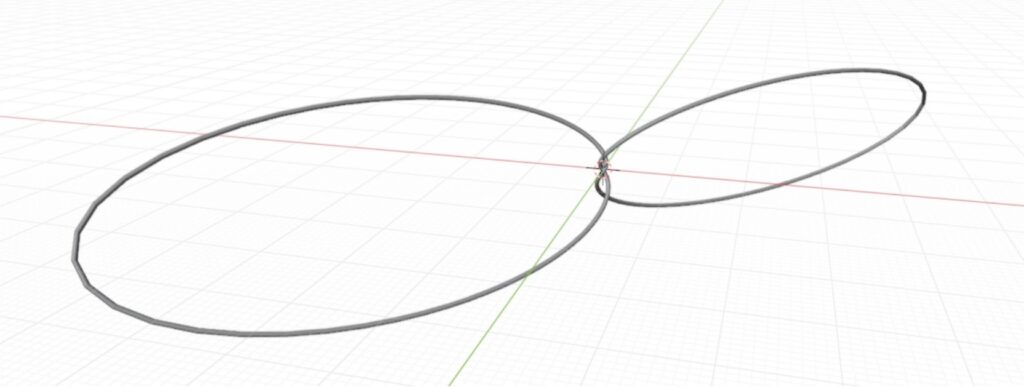# Rolling Knots

Written by Leon-Josip Dzojic, Matilde Sciortino, and Pirmin Kupffer

In the year 1980 M. H. Freedman conjectured that every generic smooth knot in three dimensional Euclidean space has a tritangent plane, i.e., a plane tangent to three distinct points. However, ten years later, H. R. Morton found a parametrization of trefoil knots that constructs a family of counterexamples . What’s interesting about such a tritangentless knot is that when sitting on a plane, it has no stable position and is therefore able to roll!

Morton constructed the trefoil knots as $$(3,2)$$ curves on a torus by stereographic projection from the 3-sphere. He starts with a trefoil knot $$T \subset S^{3}$$, embedded parametrically as

$$(a \cos 3 \theta, a \sin 3 \theta, b \cos 2 \theta, b \sin 2 \theta)\, ,$$

with $$a^{2}+b^{2}=1, a, b \neq 0$$. He then embeds these knots in $$\mathbb{R}^{3}$$ by standard stereographic projection and is able to show that the curves are tritangentless for each choice of $$(a, b)$$.

A. Eget, S. K. Lucas and L. Taalman further numerically optimized the ability of a Morton knot to roll . The center of mass of such a knot is at its center in any orientation, due to its symmetry. This center rises and falls as the knot rolls across a flat surface. Therefore, the smaller the vertical variation of this center of mass, the less energy needs to be transferred between kinetic and potential, which allows for a longer roll. As one varies the above mentioned parameter $$a$$, one obtains different amplitudes of oscillation in the vertical direction of the center of mass. Eget, Lucas and Taalman consequently calculated the vertical variation of the center of mass and scaled it by the average height of the knot, to then find the best choice for the parameter $$a$$. In our project, we developed this idea further. We calculated the relative variation of the center of mass’ velocity in the direction of rolling and optimized this function instead.

Eget, Lucas and Taalman also introduced a $$z$$-scaling factor that varies the center of mass locations when the knot rolls. For any given value of $$a$$ one can then choose the $$z$$-scaling factor that minimizes the vertical variation of the center of mass. Moreover, we introduced a third parameter, therefore looking for the “best-rolling” Morton knot within the following larger family of knots:

$$x(t)=\frac{ a \cos (3 t)}{sgpp-b \sin (2 t)}, \quad y(t)=\frac{ a \sin (3 t)}{sgpp-b \sin (2 t)}, \quad z(t)=\frac{z b \cos (2 t)}{sgpp-b \sin (2 t)},$$

for $$0 \leq t \leq 2 \pi$$, with $$a,b,z > 0, a^{2}+b^{2}=1, sgpp > b$$.

The minimization of both the vertical and the transversal displacement leads to degenerate knots which tend to stretch and become thinner and thinner, so we can’t find a global optimum of the 2-parameter optimization that is suitable to be 3D printed. Nevertheless, we could find knots that roll better than the “baseline knot” of Eget, Lucas and Taalman’s paper, as you can see in the following image (where $$dV$$ stands for vertical displacement and $$dT$$ the transversal one).(a) $$(0.5831, 1.0)$$, $$dV < 2\%, dT \approx 32\%$$(b) $$(0.3831, 0.6)$$, $$dV < 1\%, dT \approx 20\%$$

The 3-parameter optimization doesn’t provide better solutions (when adjusted for rolling distance), but it can help improving the the roll-ability of a knot given by fixed, “bad” $$a$$ and $$z$$ values.Morton knots tend to degenerate when optimized for roll-ability (here: $$(0.1473, 0.2225)$$, $$dV < 0.2\%, dT < 8\%$$).

As physical 3D printed models, these knots are beautiful kinetic objects that roll much more smoothly than one would guess when only looking at them.

References:

1. Hugh Morton. Trefoil knots without tritangent planes. Bulletin of The London Mathematical Society, 23:78–80, 01 1991.
2. Abigail Eget, Stephen Lucas, and Laura Taalman. Optimizing morton’s tritangentless knots for rolling. 08 2020.